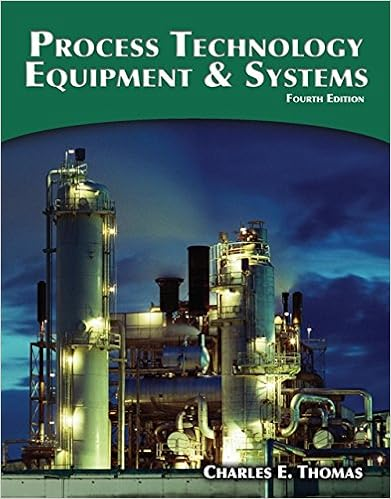# CHM 111 Properties of Gases Sp16(1) - PROPERTIES OF GASES...

• Lab Report
• 11
• 0% (2) 0 out of 2 people found this document helpful

This preview shows page 1 - 2 out of 11 pages.

##### We have textbook solutions for you!
The document you are viewing contains questions related to this textbook.The document you are viewing contains questions related to this textbook.
Chapter 14 / Exercise 12
Process Technology Equipment and Systems
ThomasExpert Verified
1 | Experiment: Properties of Gases PROPERTIES OF GASES OBJECTIVESPART A : CHARLES’S LAWTo observe the effect of change in temperature on the volume of a gas (Charles’s Law). To use a graph of Charles’s Law to predict the value of absolute zero. PART B : DETERMINATION OF THE MOLAR MASS OF A VOLATILE LIQUID To determine the molar mass of a volatile pure liquid by applying the Ideal Gas Law calculation to a known mass of the vapor. INTRODUCTION &BACKGROUNDPart A : CHARLES’S LAWIn cold weather, the tires on a bicycle seem to go flat and a balloon seems to deflate. In hot weather, a full tire or balloon may burst. Such examples illustrate that changes in gas volume are related to changes in temperature. When the temperature increases, the kinetic energy of gas molecules increases, and the volume of the gas must expand if the pressure remains constant. When the molecules slow down as the temperature decreases, the volume must decrease. According to Charles’s Law, at constant pressure the volume of a fixed amount of gas is directlyproportional to its absolute (Kelvin) temperature. Another way of stating Charles’slaw is that the ratioof V/Tfor a gas remains constant as long as the pressure and number of moles do not change. Therefore, we can write Charles’s Law as an equality relating the initial volume/temperature ratio (V1/T1) to a second volume/temperature ratio (V2/T2). 𝐕?Charles’s Law: 𝐕𝐓?= 𝐓?(P, n constant) The gases with which we are familiar in this world possess a molecular volume and interact with other gas molecules. When dramatically cooled or compressed, these real gases eventually condense into the liquid phase, then solidify. In a hypothetical world, molecules of gases (which are referred to as idealgases) are presumed to possess negligible volume and have no attractive forces between them. These gases possess the volume of their container, and according to Charles’s Law, their volumes decrease linearly with decreasing temperature. When we extrapolate these relationships, they all converge to one temperature, -273.15 ˚C, where the volume of each gas is presumably zero. For that reason, it is rational to define a temperature scale in which the lowest possible temperature is defined as zero; this is the absolute, or Kelvin temperature scale. On the Kelvin scale, this low temperature is termed absolute zero(0 K) and is -273.15 degrees on the Celsius scale. Although we can never reach absolute zero, scientists have achieved temperatures near 1 x 10-10K. In this experiment, the volume of a gas (air) will be measured at several different temperatures at which the gas approximates ideal behavior. Using the data, a graph of the relationship between volume and temperature will be prepared, and the value of absolute zero will be predictedby extending the graph line (extrapolating) to the axis where the volume of gas would theoretically decrease to zero.
?
##### We have textbook solutions for you!
The document you are viewing contains questions related to this textbook.The document you are viewing contains questions related to this textbook.
Chapter 14 / Exercise 12
Process Technology Equipment and Systems
ThomasExpert Verified
•••